Definition: multiplication from The Penguin Dictionary of Science

A fundamental operation in arithmetic in which two numbers a and b are combined to give a third, their product, written as a × b or a•b or ab. Initially defined by repeated addition of whole numbers, so that 2 × 3 means ‘2 lots of 3’, multiplication is successively extended to fractions and to all real and complex numbers. Algebraic expressions are multiplied in accordance with the ➤distributive law.

Summary Article: Lesson 1-4: Multiplication
From Homework Helpers: Basic Math and Pre-Algebra

Multiplication is a shortcut for addition. The expression 4 × 5 can be interpreted as either 5 + 5 + 5 + 5 or 4 + 4 + 4 + 4 + 4. In other words, 4 × 5 can be thought of as adding four fives or as adding five fours. Being familiar with the multiplication table (or times table) for the first 10 whole numbers will be very helpful in almost every math class you take.

There are three popular symbols used to represent multiplication: ×, ·, and ()(). The expressions 4 × 5, 4 · 5, and (4)(5) all mean the same thing. The numbers that are being multiplied together are called factors, and the result is called the product. With multiplication the order doesn't matter; evaluating 4 × 5 gives the same result as evaluating 5 × 4. We say that multiplication is commutative (just as addition is).

Multiplying by 10, 100, 1,000, etc. is probably the nicest multiplication of all. Notice that 6 × 10 = 60, 6 × 100 = 600, and 6 × 1,000 = 6,000. There is a pattern worth noting: When multiplying a number by 10 you just take that number and put a 0 at the end. When multiplying a number by 100, you just take that number and put two 0s at the end. When multiplying a number by 1,000, you just take that number and put three 0s at the end.

Sometimes multiplication problems are written horizontally as shown here: 4 × 5 = 20. Other times, multiplication is written vertically: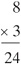The vertical representation is useful when a multiplication problem involves one or more two-digit numbers.

Example 1

Find the product: 15 × 6

Solution: First find the product 5 × 6, which is 30. Put a 0 in the ones', place of the product and carry over 3 tens. Then, find the product 1 × 6, which is 6, and add the 3 tens that you carried over to get 9: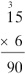Example 2

Find the product: 26 × 4

Solution: First, find the product 6 × 4, which is 24. Put a 4 down in the ones' place and carry over the 2 tens. Then, find the product 2 × 4, which is 8, and add the 2 tens that you carried over to get 10: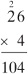To multiply two two-digit numbers together, break the problem up into two problems that involve multiplying one two-digit number and one one-digit number, and add the two results together. There's just one twist: When you work out the second product, you must shift your numbers to the left by putting a 0 in the ones' place. This is because the second number is in the tens' place; you've already taken care of the ones' place with the first product. This method will become clearer once I discuss the distributive property.

Example 3

Find the product: 32 × 43

Solution: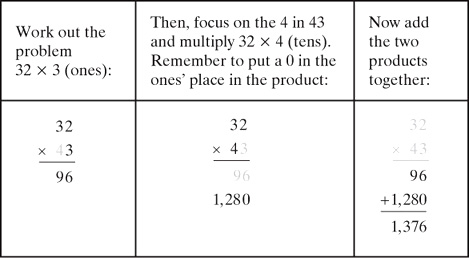So 32 × 43 = 1,376.

Example 4

Find the product: 68 × 23

Solution: Break the problem up into two products: 68 × 3 (ones) and 68 × 2 (tens) then add the results: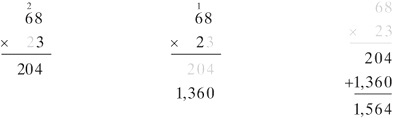So 68 × 23 = 1,564.

When you multiply 3 numbers together, the order in which you perform the multiplication doesn't matter. For example, when finding the product 4 × 2 × 5, it doesn't matter if you first find 4 × 2 and then multiply the result by 5 · (4 × 2) × 5 = 8 × 5 = 40 or if you first find 2 × 5 and then multiply the result by 4 · 4 × (2 × 5) = 4 × 10 = 40. Multiplication is said to be associative (again, just as addition is). It doesn't matter how you group a string of products together, or which products you find first.

Lesson 1-4 Review

Find the following products.

1. 4 × 9

2. 36 × 8

3. 62 × 18

4. 3 × 5 × 8

5. 16 × 10,000Copyright 2011 © by Denise Szecsei

Related Articles

Full text Article identity element
Merriam-Webster's Collegiate(R) Dictionary

(1902) : an element (as 0 in the set of all integers under addition or 1 in the set of positive integers under multiplication) that leaves any eleme

Full text Article multiplication
The Penguin English Dictionary

/multiplikaysh(ə)n/ noun 1 the act or process or an instance of multiplying or being multiplied. 2 a mathematical operation...

Full text Article distributive1
The Penguin English Dictionary

/distribyootiv/ adj 1 relating to or concerned with distribution. 2 in grammar, denoting a pronoun or determiner such as ...

See more from Credo